# Attenuator Math

This page is in response to the following question: how do you solve attenuator equations?

It's so simple that even a 47-year-old can do it, using Ohm's Law! We'll look at one example here, then you are all on your own. If you can't do this math, you need to consider a new career, perhaps in your company's Six Sigma organization. The only "formula" you have to remember is that resistors in parallel have a net value of the product over the sum.

Start by drawing a picture of the attenuator, and include the voltage source and its impedance. Remember to use "2V" for the voltage source, and Z0 for its impedance. Then under the matched condition, ZIN and Z0 will form a 50% voltage divider and the load will get the maximum power V^2/Z0.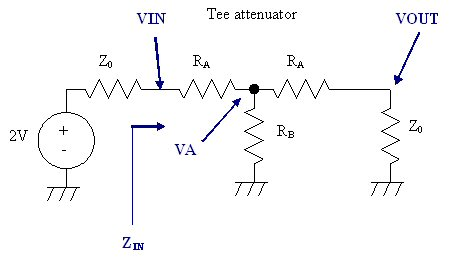Now, let's start by calculating the attenuation, which is given by Vout/Vin. What you have is a two-step voltage divider. We can write two equations from this: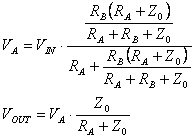Note that if you work on the above expressions you can solve for VIN/VOUT in terms of RA, RB and Z0.

An important property of the attenuator is that ZIN must be matched to Z0. This gives the following expression: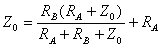More coming!

### Effect of sheet resistance variation on attenuators

Let's jump ahead to a common attenuator problem. What happens when you are unable to laser trim the resistors in a tee or pi attenuator to 1% values, when you are making thin-film attenuators? This is often the case, because the way the network is hooked up it is hard to separate R1 from R2, etc.

It turns out attenuators are a lot more forgiving than you'd think, because of the combination of errors in series and shunt elements tends to cancel each other out.

Yes, we will post the equations for this soon... but we checked our answers against an ADS model, so we are confident that the plots are correct.

Below is a plot showing the attenuation error for either a tee or a pi, when sheet resistance is varied from 0.6, 0.8 and 0.9 of its nominal value. By the way, it makes no difference if you use a tee or a pi, the error you create when sheet resistance drifts is exactly the same. If you are off the mark in the opposite direction (Rsh=1.66, 1.25 and 1.11 of nominal) you get the same errors. The sheet resistance has to be 40 percent low (or 66% high) to make the error more than 0.55 dB. Sweet!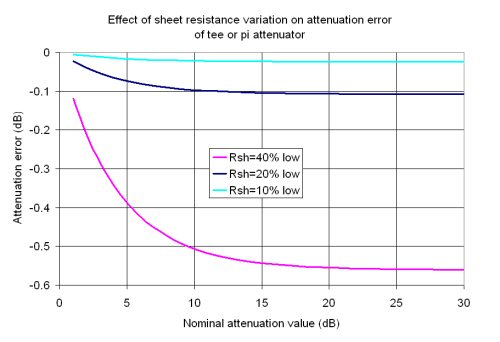Here's a look at the input return loss with the same sheet resistance errors. Here even if you are under the nominal by 40%, you still get 12 dB return loss.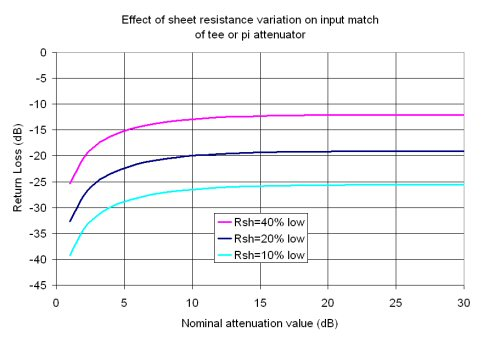More to come!

Author : Unknown Editor